Courses

# Physics Test 5 - Magnetic Effect, Magnetism, Electromagnetic Induction And Alternating Current

## 30 Questions MCQ Test JEE Main Mock Test Series 2020 & Previous Year Papers | Physics Test 5 - Magnetic Effect, Magnetism, Electromagnetic Induction And Alternating Current

Description
This mock test of Physics Test 5 - Magnetic Effect, Magnetism, Electromagnetic Induction And Alternating Current for JEE helps you for every JEE entrance exam. This contains 30 Multiple Choice Questions for JEE Physics Test 5 - Magnetic Effect, Magnetism, Electromagnetic Induction And Alternating Current (mcq) to study with solutions a complete question bank. The solved questions answers in this Physics Test 5 - Magnetic Effect, Magnetism, Electromagnetic Induction And Alternating Current quiz give you a good mix of easy questions and tough questions. JEE students definitely take this Physics Test 5 - Magnetic Effect, Magnetism, Electromagnetic Induction And Alternating Current exercise for a better result in the exam. You can find other Physics Test 5 - Magnetic Effect, Magnetism, Electromagnetic Induction And Alternating Current extra questions, long questions & short questions for JEE on EduRev as well by searching above.
QUESTION: 1

Solution:
QUESTION: 2

### A closely wound flat circular coil of 25 turns of wire has diameter of 10 cm which carries current of 4 A, the flux density at the centre of a coil will be

Solution:

Flux density = 2 * π * N * i/10r
or,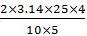= 12.56G or 1.25 * 10-3T

QUESTION: 3

### An electron is travelling along the x-direction. It encounters a magnetic field in the y-direction. Its subsequent motion will be

Solution: Because a force of q(v×B) is acted on e⁻ toward +z axis that will change the direction of e⁻ and then it will move in a circle in x-z plane
QUESTION: 4
The magnetic field due to a straight conductor of uniform cross-section of radius a and carrying a steady current is represented by
Solution:
QUESTION: 5

A cyclotron is operating at a frequency of  12 * 106 Hz. Mass of deuteron is 3.3 * 10-27 kg  and charge on deuteron is 1.6 * 10-19  induction of the neccessary magnetic field is

Solution:
QUESTION: 6

The strength of the transverse magnetic field required to bend photoelectrons of maximum kinetic energy with in a circle of radius 50 cm when light of wavelength 4000Å is incident on barium emitter is (work function of barium is 2.5 eV)

Solution:

key idea: Body performing circular motion is always acted upon by a centripetal force provided by magnetic field. If the kinetic energy of photoelectrons emitted from the metal surface is E1 and W the work function them from Einstein's photoelectric equation, we have Eh = hv -W where v is frequency and h the Planck's constant
∴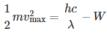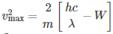Putting the numercial values from the question, we have
∴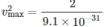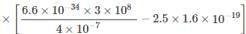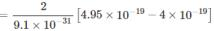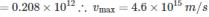A body performing circular motion is acted upon by a force directed towards its centre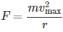Also the magnetic force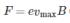On equating.
We have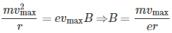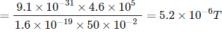QUESTION: 7
The semicircular portion (radius R) of a wire carrying current i is placed in a uniform magnetic field B. The magnetic field is perpendicular to the plane of the circle. The magnetic of the force on the wire is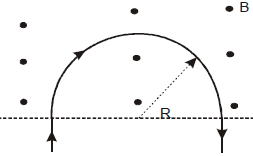Solution:
QUESTION: 8
A & B are two concentric circular conductors of centre O and carrying currents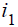and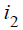as shown in the diagram. If the ratio of their radii is 1:2 and ratio of the flux densities at O due to A and B is 1:3, then the value of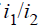will be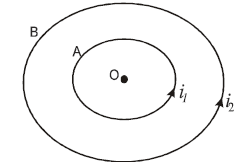Solution:
QUESTION: 9
Domain formation is the necessary feature of
Solution:
QUESTION: 10
The direction of the null points is on the equation line of a bar magnet, when the north pole of the magnet is pointing
Solution:
QUESTION: 11
Two magnets each of magnetic moment M are placed so as to form a cross at right angles to each other. The magnetic moment of the system will be
Solution:
QUESTION: 12

At a certain place, the horizontal component of earth’s magnetic field is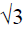times of its vertical component. the angle of dip at that place is

Solution:
QUESTION: 13

A magnet makes 30 oscillations per minute in the earth’s magnetic field. If the magnetic field is doubled. The period of oscillation of magnet is

Solution: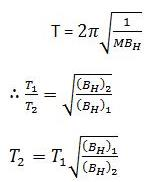n1 = 30 oscillation/min
n1 = 1/2  oscillation/s
T1 = 2s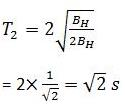QUESTION: 14
A moving conductor coil produces an induced emf. This is in accordance with
Solution:
QUESTION: 15
The varying current in a coil changes from 10 A to zero in 0.5 s. If the average emf induced in the coil is 220 V, then the self-inductance of the coil will be
Solution: Avg E= L(∆i/∆t) 220=L (10/0.5) 220=L(20) L=11H
QUESTION: 16
Two circular coils can be arranged in any of the three situations shown in the figure. Their mutual inductance will be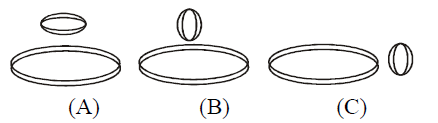Solution:
QUESTION: 17

In the circuit shown in the figure, what is the value of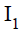just after pressing the key K?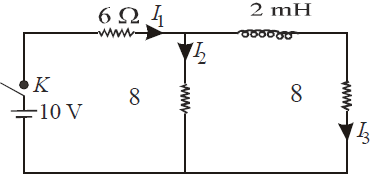Solution:
QUESTION: 18
A uniform but time-varying magnetic field B(t) exists in cylindrical region of radius a and is directed into the plane of the paper, as shown. The magnitude of the induced electric field at point P at a distance r from the centre of the circular region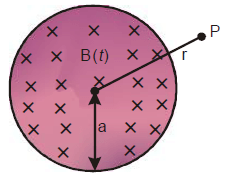Solution:
Construct a concentric circle of radius r. The induced electric field (E) at any point on the circle is equal to that at P. For this circle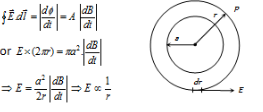QUESTION: 19
A device for generating and alternating current of a desired frequency is known as
Solution:
QUESTION: 20
In an AC circuit containing only capacitance, the current
Solution:
QUESTION: 21

The rms value of emf of a saw toothed AC of peak voltage 60 volt is

Solution:
QUESTION: 22
A horizontal rod kept in east-west direction falls at a place other than poles. Which end of the rod will acquire positive polarity
Solution:
QUESTION: 23

Two coils C1 & C2  are placed adjacent to each other, if current is changed in coil C1  at a rate of 5A/sec, the emf induced in coil C2 is 20mV. What emf will be induced in 7C1 if the current is changed in Coil Cat a rate of 7 A/Sec (Assume there is no change in orientation or position of the coils)

Solution:
QUESTION: 24
A magnetic needle is kept in a non uniform magnetic
field. It experiences
Solution:
QUESTION: 25

Direction : Read the following question and choose

A. If both Assertion and Reason are correct and reason is the correct explanation of assertion

B. If both Assertion and Reason are true, but Reason is not correct explanation of the Assertion

C. If Assertion is true, but the Reason is false

D. If Assertion is false, but the Reason is true

E. If both assertion and reason are false

Assertion : A.C. is more dangerous than D.C.
Reason: The peak value of A.C. is 41.4% higher than the D.C.

Solution:
QUESTION: 26

Direction : Read the following question and choose

A. If both Assertion and Reason are correct and reason is the correct explanation of assertion

B. If both Assertion and Reason are true, but Reason is not correct explanation of the Assertion

C. If Assertion is true, but the Reason is false

D. If Assertion is false, but the Reason is true

E. If both assertion and reason are false

Assertion: Self Inductance is also known as inertia of electricity.
Reason : Self Inductance is the property of Coil due to which the coil opposes the flow current through it

Solution:
QUESTION: 27

Direction : Read the following question and choose

A. If both Assertion and Reason are correct and reason is the correct explanation of assertion

B. If both Assertion and Reason are true, but Reason is not correct explanation of the Assertion

C. If Assertion is true, but the Reason is false

D. If Assertion is false, but the Reason is true

E. If both assertion and reason are false

Assertion: In the phenomenon of mutual induction self induction of each of the coils persists
Reason: Self induction arises when strength of current in one coil changes. In this case, current may be changing in both the individual coils

Solution:
QUESTION: 28

Direction : Read the following question and choose

A. If both Assertion and Reason are correct and reason is the correct explanation of assertion

B. If both Assertion and Reason are true, but Reason is not correct explanation of the Assertion

C. If Assertion is true, but the Reason is false

D. If Assertion is false, but the Reason is true

E. If both assertion and reason are false

Assertion: It is more difficult to push a magnet into a coil with more loops
Reason : This is because emf induced in each current loop resists the motion of the magnet

Solution:
QUESTION: 29

Direction : Read the following question and choose

A. If both Assertion and Reason are correct and reason is the correct explanation of assertion

B. If both Assertion and Reason are true, but Reason is not correct explanation of the Assertion

C. If Assertion is true, but the Reason is false

D. If Assertion is false, but the Reason is true

E. If both assertion and reason are false

Assertion : Xm–T graph for a diamagnetic material is a straight line parallel to T-axis
Reason : This is because susceptibility of a diamagnetic material is not affected by temperature

Solution:
QUESTION: 30

Direction : Read the following question and choose

A. If both Assertion and Reason are correct and reason is the correct explanation of assertion

B. If both Assertion and Reason are true, but Reason is not correct explanation of the Assertion

C. If Assertion is true, but the Reason is false

D. If Assertion is false, but the Reason is true

E. If both assertion and reason are false

Assertion : In an electric field, an electron beam perpendicular to field is deflected along a parabola, and in a magnetic field, the electron path perpendicular to the field will be circular
Reason: In electric field, force is perpendicular to path and along the field.In magnetic field, force is perpendicular to both, the magnetic field and path of beam

Solution: